Mathematical documents include elements that require special formatting and numbering such as theorems, definitions, propositions, remarks, corollaries, lemmas and so on. This article explains how to define these environments in LaTeX. Contents 1 Introduction 2 Numbered theorems, definitions, corollaries and lemmas 3 Unnumbered theorem-like environments 4 Theorem styles 5 Proofs 6 Changing the qed symbol 7 Reference guide 8 Further reading Introduction Numbered environments in LaTeX can be defined by means of the command \newtheorem. An example is presented below: \documentclass{article} \usepackage[utf8]{inputenc} \usepackage[english]{babel} \newtheorem{theorem}{Theorem} \begin{document} \section{Introduction} Theorems can easily be defined \begin{theorem} Let $f$ be a function whose derivative exists in every point, then $f$ is a continuous function. \end{theorem} \end{document}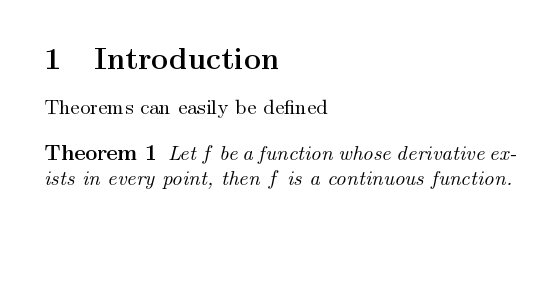The command \newtheorem{theorem}{Theorem} has two parameters, the first one is the name of the environment that is defined, the second one is the word that will be printed, in boldface font, at the beginning of the environment. Once this new environment is defined it can be used normally within the document, delimited it with the marks \begin{theorem} and \end{theorem}.   Open an example in Overleaf Numbered theorems, definitions, corollaries and lemmas The numbering of the environments can be controlled by means of two additional parameters in the \newtheorem command. Let's see: \documentclass{article} \usepackage[utf8]{inputenc} \usepackage[english]{babel} \newtheorem{theorem}{Theorem}[section] \newtheorem{corollary}{Corollary}[theorem] \newtheorem{lemma}[theorem]{Lemma} \begin{document} \section{Introduction} Theorems can easily be defined \begin{theorem} Let $f$ be a function whose derivative exists in every point, then $f$ is a continuous function. \end{theorem} \begin{theorem}[Pythagorean theorem] \label{pythagorean} This is a theorema about right triangles and can be summarised in the next equation $x^2 + y^2 = z^2$ \end{theorem} And a consequence of theorem \ref{pythagorean} is the statement in the next corollary. \begin{corollary} There's no right rectangle whose sides measure 3cm, 4cm, and 6cm. \end{corollary} You can reference theorems such as \ref{pythagorean} when a label is assigned. \begin{lemma} Given two line segments whose lengths are $a$ and $b$ respectively there is a real number $r$ such that $b=ra$. \end{lemma}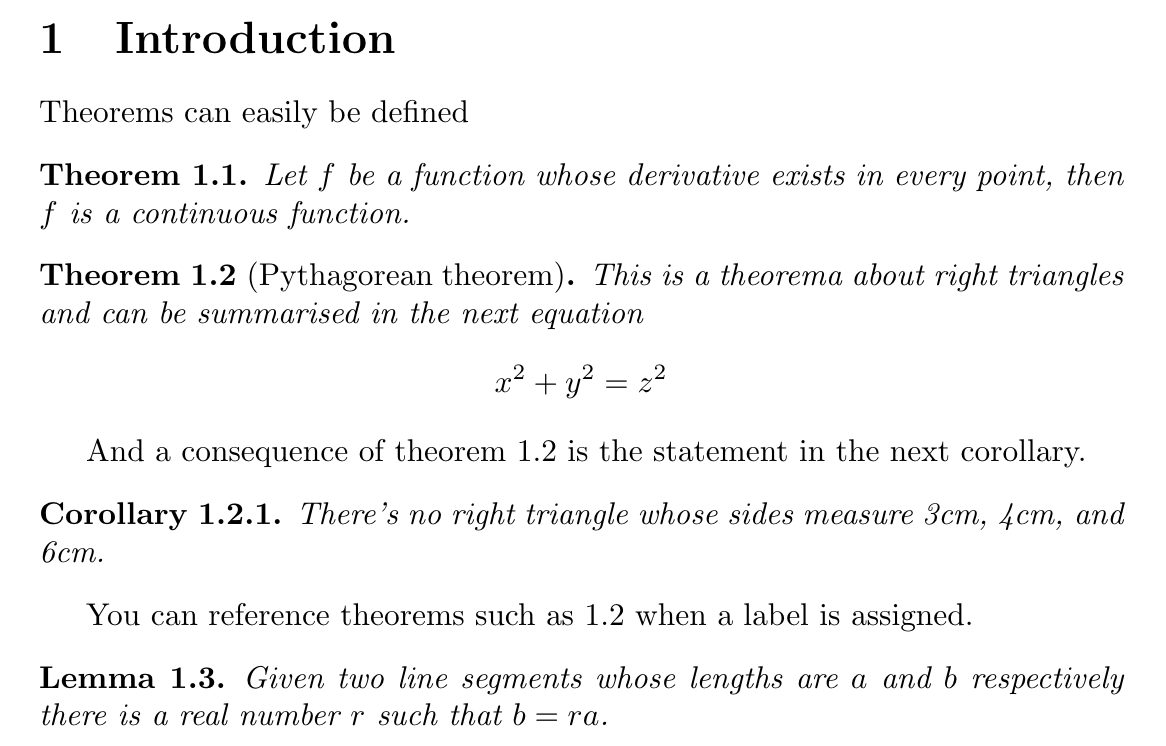There are three new environments defined in the preamble. \newtheorem{theorem}{Theorem}[section] This is the example presented in the introduction but it has the additional parameter [section] that restarts the theorem counter at every new section. \newtheorem{corollary}{Corollary}[theorem] An environment called corollary is created, the counter of this new environment will be reset every time a new theorem environment is used. \newtheorem{lemma}[theorem]{Lemma} In this case, the even though a new environment called lemma is created, it will use the same counter as the theorem environment. Some famous theorems have their own names, for these cases you can add said name inside brackets in the environment opening command. In the example the line \begin{theorem}[Pythagorean theorem] prints "Pythagorean theorem" at the beginning of the paragraph. As with many other numbered elements in LaTeX, the command \label can be used to reference theorem-like environments within the document.   Open an example in Overleaf Unnumbered theorem-like environments Sometimes it becomes handy to have an unnumbered theorem-like environments to add remarks, comments or examples to a mathematical document. The package amsthm provides this functionality. \documentclass{article} \usepackage[utf8]{inputenc} \usepackage[english]{babel} \usepackage{amsthm} \newtheorem*{remark}{Remark} \begin{document} Unnumbered theorem-like environments are also posible. \begin{remark} This statement is true, I guess. \end{remark} \end{document}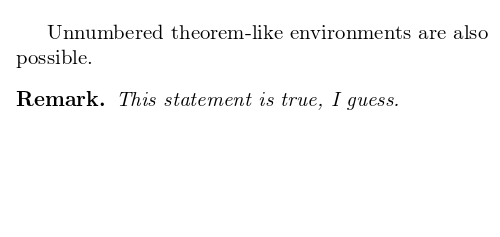The syntax of the command \newtheorem* is the same as the non-starred version, except for the counter parameters. In this example a new unnumbered environment called remark is created.   Open an example in Overleaf Theorem styles A feature that is important when working in a mathematical document is to easily tell apart, say, definitions from theorems by its formatting. The package amsthm provide special commands to accomplish this. \documentclass{article} \usepackage[utf8]{inputenc} \usepackage[english]{babel} \usepackage{amsthm} \theoremstyle{definition} \newtheorem{definition}{Definition}[section] \theoremstyle{remark} \newtheorem*{remark}{Remark} \begin{document} Unnumbered theorem-like environments are also possible. \begin{remark} This statement is true, I guess. \end{remark} And the next is a somewhat informal definition \begin{definition}[Fibration] A fibration is a mapping between two topological spaces that has the homotopy lifting property for every space $X$. \end{definition} \end{document}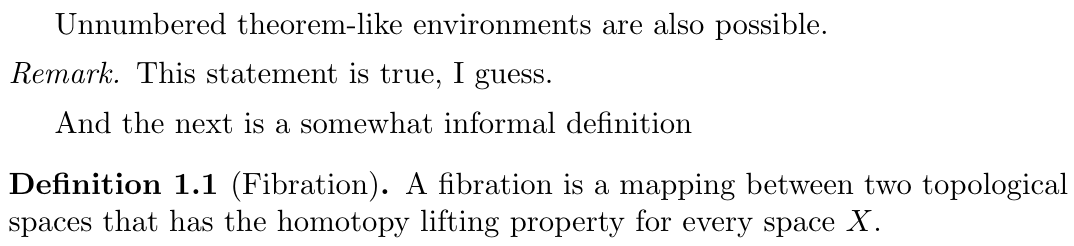The command \theoremstyle{ } sets the styling for the numbered environment defined right below it. In the example above the styles remark and definition are used. Notice that the remark is now in italics and the text in the environment uses normal (Roman) typeface, the definition on the other hand also uses Roman typeface for the text within but the word "Definition" is printed in boldface font. See the reference guide for more theorem styles.   Open an example in Overleaf Proofs Proofs are the core of mathematical papers and books and is customary to keep them visually apart from the normal text in the document. The package amsthm provides the environment proof for this. \documentclass{article} \usepackage[utf8]{inputenc} \usepackage[english]{babel} \usepackage{amsthm} \newtheorem{theorem}{Theorem}[section] \newtheorem{lemma}[theorem]{Lemma} \begin{document} \begin{lemma} Given two line segments whose lengths are $a$ and $b$ respectively there is a real number $r$ such that $b=ra$. \end{lemma} \begin{proof} To prove it by contradiction try and assume that the statement is false, proceed from there and at some point you will arrive to a contradiction. \end{proof} \end{document}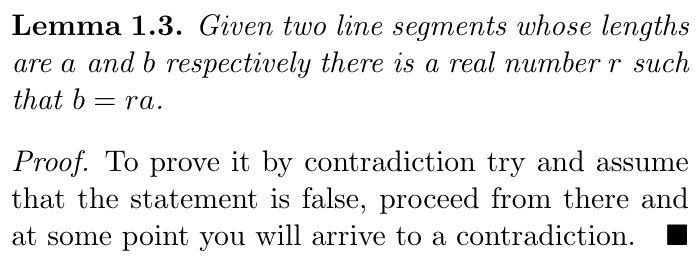The word Proof is italicized and there is some extra spacing, also a special symbol is used to mark the end of the proof. This symbol can be easily changed, to learn how see the next section.   Open an example in Overleaf Changing the qed symbol To change the symbol printed at the end of a proof is straightforward. \documentclass{article} \usepackage[utf8]{inputenc} \usepackage[english]{babel} \usepackage{amsthm} \usepackage{amssymb} \newtheorem{theorem}{Theorem}[section] \newtheorem{lemma}[theorem]{Lemma} \renewcommand\qedsymbol{$\blacksquare$} \begin{document} \begin{lemma} Given two line segments whose lengths are $a$ and $b$ respectively there is a real number $r$ such that $b=ra$. \end{lemma} \begin{proof} To prove it by contradiction try and assume that the statemenet is false, proceed from there and at some point you will arrive to a contradiction. \end{proof} \end{document}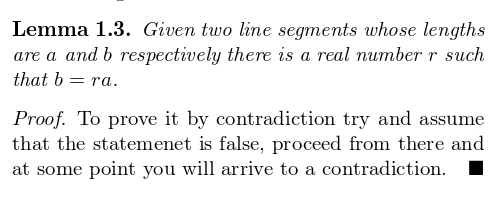The command \renewcommand\qedsymbol{$\blacksquare$} changed the default white square for a black square that is printed by $\blacksquare$, the parameter inside the braces. You can change this for any other symbol or text, for instance you can use \renewcommand\qedsymbol{QED} To print the traditional QED (quod erat demonstrandum) at the end of a proof.   Open an example in Overleaf Reference guide Theorem styles definition boldface title, romand body. Commonly used in definitions, conditions, problems and examples. plain boldface title, italicized body. Commonly used in theorems, lemmas, corollaries, propositions and conjectures. remark italicized title, romman body. Commonly used in remarks, notes, annotations, claims, cases, acknowledgments and conclusions. Further reading For more information see: Mathematical expressions Environments Display style in math mode List of Greek letters and math symbols Mathematical fonts Multi-file LaTeX projects Cross referencing sections and equations Hyperlinks The amsthm package documentation